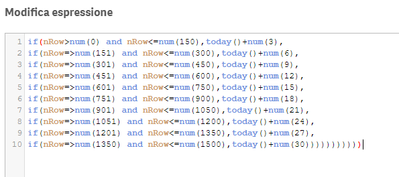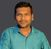# New to Qlik Sense

If you’re new to Qlik Sense, start with this Discussion Board and get up-to-speed quickly.

Announcements
cancel
Showing results for
Did you mean:Contributor III

## error in expression, where?

hello to all,

how can halo me to find why qlik show me the error below?

error in expression: ')' axpected1 Solution

Accepted SolutionsSpecialist

Hi

You used wrong operator ( =>) it is >=

try below

=if(nRow>num(0) and nRow <=num(150),Today()+num(3),
if(nRow>=num(151) and nRow <=num(300),Today()+num(6),
if(nRow>=num(301) and nRow <=num(450),Today()+num(9),
if(nRow>num(451) and nRow <=num(600),Today()+num(12),
if(nRow>=num(601) and nRow <=num(750),Today()+num(15),
if(nRow>=num(751) and nRow <=num(900),Today()+num(18),
if(nRow>=num(901) and nRow <=num(1050),Today()+num(21),
if(nRow>=num(1051) and nRow <=num(1200),Today()+num(24),
if(nRow>=num(1201) and nRow <=num(1350),Today()+num(27),
if(nRow>=num(1351) and nRow <=num(1500),Today()+num(30)))))))))))Specialist

Hi

You used wrong operator ( =>) it is >=

try below

=if(nRow>num(0) and nRow <=num(150),Today()+num(3),
if(nRow>=num(151) and nRow <=num(300),Today()+num(6),
if(nRow>=num(301) and nRow <=num(450),Today()+num(9),
if(nRow>num(451) and nRow <=num(600),Today()+num(12),
if(nRow>=num(601) and nRow <=num(750),Today()+num(15),
if(nRow>=num(751) and nRow <=num(900),Today()+num(18),
if(nRow>=num(901) and nRow <=num(1050),Today()+num(21),
if(nRow>=num(1051) and nRow <=num(1200),Today()+num(24),
if(nRow>=num(1201) and nRow <=num(1350),Today()+num(27),
if(nRow>=num(1351) and nRow <=num(1500),Today()+num(30)))))))))))